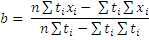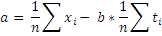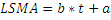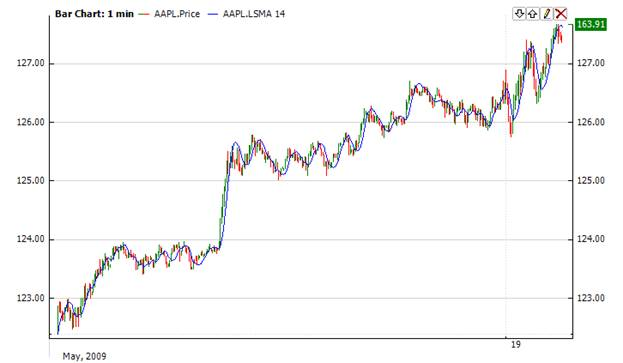﻿Least Squares Moving Average

# Least Squares Moving Average

The Least Squares Moving Average (Lsma) first calculates a least squares regression line over the preceding time periods, and then projects it forward to the current period.  In essence, it calculates what the value would be if the regression line continued.Market Signals

Lsma gives trading signals like any moving average – if Lsma line crosses price from above it is a buy signal and vice versa.Calculationwhere x is the price, t is the time of this price, n is the periodChart ExampleImplementation and Usage

To initialize Lsma indicator, use one of the following constructors:

Lsma – sets default values: period = 14, useTime = false.

Lsma(Int32, Boolean) – sets period and useTime flag.  If this flag is true, to calculate the indicator values, time (in 100 nanoseconds) will be used and you need to use method Add() with time.  If this flag is false, a number of data points will be used for calculation.  In this case you can use method Add() without time.

Lsma(TimeSpan, Boolean) – sets time period and useTime flag.

Use

LSMA - property to get current value.Example
C#
``` 1// Create new instance
2Lsma lsma = new Lsma(20, false);
3
4// Number of stored values
5lsma.HistoryCapacity = 2;
6